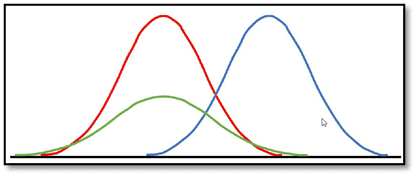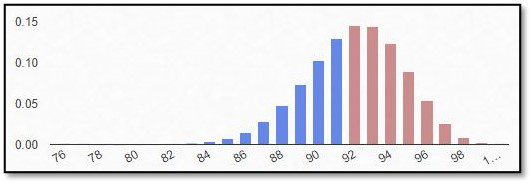# What is the normal distribution?The normal distribution or Gauß distribution is called normal, as it is seen as the ideal case of a distribution. It is showing the distribution of the probability of an observation to occur in a certain class, for metric data.

The characteristics of the normal distribution are the following: it has the shape of a bell jar and is symmetrical, it has its maximum in µ and its inflection points in µ minus one standard deviation as well as plus one standard deviation, towards infinity and minus infinity it approximates the x-axis.

The normal distribution is defined by two parameters: the mean, and the variance. The simplest case of a normal distribution is the standard normal distribution, where µ=0 and the standard deviation is 1 (red curve). If the mean is different from one, the whole curve is shifted accordingly along the x-axis (blue line). If the variance is larger, the curve becomes flatter and wider (green line).

One of the most important property of the normal distributed variables is that they can be transformed into the standard normal distribution by standardization, which includes two steps: subtracting the mean (x-µ) and dividing by the standard deviation. The advantage of the standard normal distribution is that it is exactly known which probabilities can be found in which ranges, regarding the area under the curve (cumulative distribution function). The values within one standard deviation around the mean (positive and negative), which is also called sigma, account for 68.27% of the data. Within the reach of two standard deviations, we will find 95.45%, and within three standard deviations we are covering 99.73% of the data. This is also known as the 68-95-99.7-rule or 3-sigma-rule.The other way around, we could also ask in which interval do 80%, 90% and 95% of the data lie, since these are the most common values in the analysis of clinical trial data. The interval around µ is 1.28, 1.64 and 1.96 sigma respectively, when the cutoff is always applied on both sides.

If you are interested in a visual presentation of this topic, please feel free to follow us on our GCP Mindset YouTube channel! If you would like to know more about how we could implement statistics in your clinical trial, send us a mail to statistics@gcp-service.com.

## More To ExploreBiostatistics

### What is the binominal distribution?

If an outcome variable that can be specified in two response possibilities only, data can be analyzed as binary based on a binomial distribution, where each observation of a defined number of “experiments” represents one of the two outcomes, and the probability of success is the same for each observation.
In this part of our blog series accompanying our #video #series “5 Minutes Statistics for Clinical Trials” we explain what a #binomial #distribution reflects and what are its characteristics.